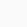# How to represent psi(𝜓) symbol in LaTeX?

Symbol/UnicodePsi/U+03C8
Type of symbolGreek small letter
Package (requirement)No
ArgumentNo
Latex command\psi
Example\psi → 𝜓

Although psi is a Greek small letter, it has many uses in mathematics and physics as a function. Simple command for this is psi. But, this is math mode command.

\documentclass{article}
\begin{document}
$\psi(A,B,C)$
$\psi(1,2,1)$
$|\psi(\textbf{e},t)|^2$
\end{document}

Output :Scroll to Top